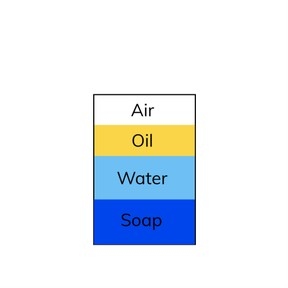Density

# Density

I can define and calculate the density for various objects.8,000 schools use Gynzy92,000 teachers use Gynzy1,600,000 students use Gynzy

## General

In this lesson, students will learn why some objects float and other objects sink in water. They will use the equation for density to determine whether objects will float or sink in water. They will also learn the equations for volume and mass.

NGSS: MS-­PS1-­4

## Learning objective

Students will be able to define and calculate the density of various objects.

## Introduction

Open the lesson with this scenario: a cargo ship that weighs over 600,000 tons floats while a rock that weighs less than one pound sinks. Have students discuss in pairs how this is possible. Students will also review the definitions of mass and volume.

## Instruction

Define density and introduce the equation for density. With the interactive instructions in the lesson, walk students through calculating the density of a rock. Next, they will compare the density of water with the density of the rock. Show students how to rearrange the density equation to solve for volume and mass. Explain that something less dense than water will float; something denser than water will sink.

Students will observe a container (a diagram is provided) with air, oil, water, and soap. In pairs, they will discuss what they can conclude about the densities of each substance.

They will do two more problems, involving calculating the mass of a 20mL sample of mercury and identifying an unknown substance with its density.

## Quiz

Students will respond to ten multiple-choice and short answer questions.

## Closing

Students will recall:
- Why should we care about density?

Lastly, they will complete an exit slip: which objects in the aquarium have a higher density than water?

### The online teaching platform for interactive whiteboards and displays in schools

• Save time building lessons

• Manage the classroom more efficiently

• Increase student engagement